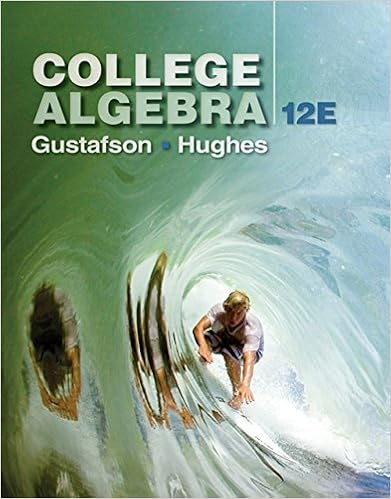# Simplifying radicals day 3 what is a perfect square

• 19

This preview shows page 4 - 9 out of 19 pages.

##### We have textbook solutions for you!
The document you are viewing contains questions related to this textbook.The document you are viewing contains questions related to this textbook.
Chapter 1 / Exercise 78
College Algebra
Gustafson/HughesExpert Verified
SIMPLIFYING RADICALS (DAY 3)What is a perfect square? ___________________________________________________What happens when you take the square root () of a perfect square (VIPS)?4
##### We have textbook solutions for you!
The document you are viewing contains questions related to this textbook.The document you are viewing contains questions related to this textbook.
Chapter 1 / Exercise 78
College Algebra
Gustafson/HughesExpert Verified
When solving quadratics, the answer is not always a perfect square when you take theof a number. In order to find the answer you will need to SIMPLIFY the of non- perfect squares.Simplify the following:1)182)-9843) -48434)12215)5026)277)9998)3239)755210)25411)16.012)50045PROCEDURE TO SIMPLIFY RADICALS (non-perfect squares):1.List perfect squares from 1 to 169 out. (VIPS) (create a list)2.Determine where the number that you are simplifying would fall in this list.3.Work UP the list to #1 to locate thelargest perfect square that goes into your number.4.Once found, break up your original (house) by placing the largest perfect squarenumber found under the first sign and place its divisor under the second sign.5.Circle the perfect square. – IT IS SO PERFECT THAT …VIPSLIST
Pythagorean Theorem Formula: _________________________________________13)Given a right triangle with leg of 8 m and a hypotenuse of 12m, determine the length of the other leg in simplest radical form.14)Given a right triangle with a hypotenuse of 28and a leg of 10. Find the length ofthe missing leg in simplest radical form.15.Given a right triangle with a leg of 8cm and a leg of 6cm. Find the length of the hypotenuse in simplest radical form.
6
LIST
11)A woman casts a shadow of 11ft. The measure from the top of her head to the tip of the shadow is 12.5ft. How tall is the woman to the nearest foot?7
12)Given a right triangle with a hyptoneuse of 29and a leg of 8, find the length of the missing leg in simplest radical form.SOLVING QUADRATICS THAT CAN’T BE FACTOREDOPTION 1: COMPLETING THE SQUARE(DAY 5)1) What is a quadratic equation?2) What are the zeros (roots) of a quadratic equation? (algebraically and graphically)
•••